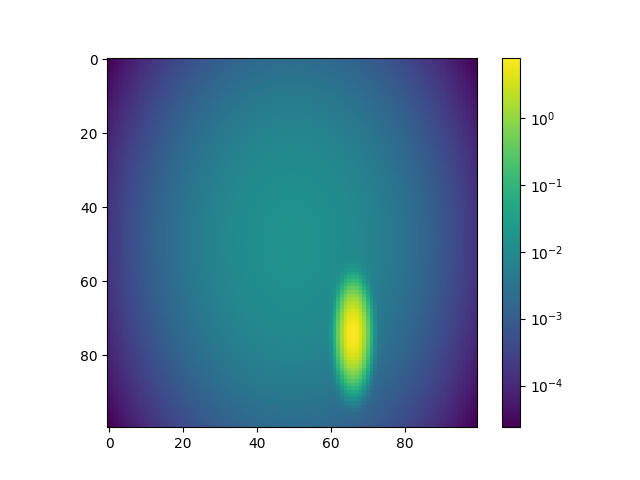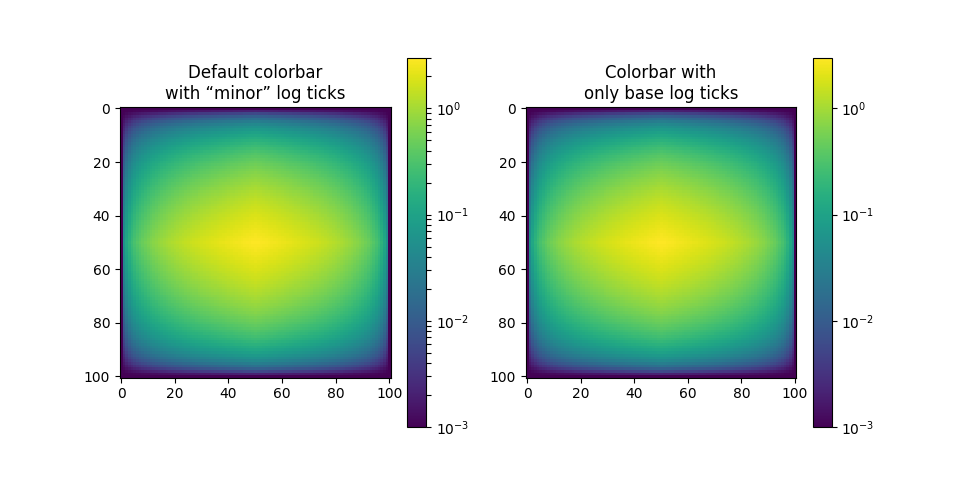# Colorbar with imshow(logNorm) shows unexpected minor ticks #8307

Closed
opened this issue Mar 16, 2017 · 9 comments

Projects
None yet

### Bug report

Bug summary

When using colorbar with imshow(image, norm=LogNorm()), the produced colorbar automatically adds minorticks, and cannot be turned off with cbar.ax.minorticks_off(). Also the font of the tick label is not the same as the font set in matplotlibrc.

Code for reproduction

```# This is modified from an example in matplotlib gallery.

import matplotlib.pyplot as plt
import numpy as np
from numpy import ma
from matplotlib import colors, ticker, cm
from matplotlib.mlab import bivariate_normal

N = 100
x = np.linspace(-3.0, 3.0, N)
y = np.linspace(-2.0, 2.0, N)

X, Y = np.meshgrid(x, y)

z = (bivariate_normal(X, Y, 0.1, 0.2, 1.0, 1.0)
+ 0.1 * bivariate_normal(X, Y, 1.0, 1.0, 0.0, 0.0))

z = ma.masked_where(z <= 0, z)

fig, ax = plt.subplots()

from matplotlib.colors import LogNorm

cs = ax.imshow(z,norm=LogNorm())

cbar = fig.colorbar(cs)

cbar.ax.minorticks_off()

plt.show()
```

Actual outcomeExpected outcome

I would expect the colorbar to not have minorticks and to use the same font as other tick labels (I set font to be Arial in matplotlibrc).

Matplotlib version

• Matplotlib version, Python version and Platform (Windows, OSX, Linux ...)

2.0.0_1, OSX

• How did you install Matplotlib and Python (pip, anaconda, from source ...)
Macports

Edit by @afvincent: removed the unnecessary instructions coming from the bug report template.

Member

### QuLogic commented Mar 17, 2017

 It appears you also have some custom rcParam settings; the ticks should not be pointing inward. In any case, I can still reproduce myself. These minor ticks are not supposed to be shown with so many major decades visible, right @efiring? @NelleV? The font weirdness is probably #8017.
Author

### billtau commented Mar 17, 2017

 Oh, yes, I changed rcParam so that so ticks point inward. Sorry I didn't mention that. First time to report something here.
Member

### NelleV commented Mar 17, 2017

 Indeed, I would not have expected minor ticks to appear.

Contributor

### afvincent commented Oct 23, 2017 • edited

 FWIW this issue still present on Matplotlib 2.1. If that was not obvious, please note that the problem does not come from `ax.minorticks_off` as the subticks are actually major ticks in the case of `LogLocator`. I did not find where is located the problem in `LogLocator` for the moment, but in the meantime a possible workaround is: ```import numpy as np import matplotlib.pyplot as plt from matplotlib.mlab import bivariate_normal from matplotlib.ticker import LogLocator from matplotlib.colors import LogNorm N = 100 x = np.linspace(-3.0, 3.0, N) y = np.linspace(-2.0, 2.0, N) X, Y = np.meshgrid(x, y) z = (bivariate_normal(X, Y, 0.1, 0.2, 1.0, 1.0) + 0.1 * bivariate_normal(X, Y, 1.0, 1.0, 0.0, 0.0)) z = np.ma.masked_where(z <= 0, z) fig, ax = plt.subplots() cs = ax.imshow(z, norm=LogNorm()) cbar = fig.colorbar(cs) # print(cbar.ax.yaxis.get_major_locator()) # => : beware, not a LogLocator instance! cbar.ax.yaxis.set_major_locator(LogLocator()) # <- Why? See above. cbar.set_ticks(cbar.ax.yaxis.get_major_locator().tick_values(z.min(), z.max())) fig.show()```Contributor

### afvincent commented Oct 24, 2017 • edited

 Well, the handling of the tick locator seems a bit convoluted with colorbars, at least for my current understanding ^^... Nevertheless, here is a workaround that is bit simpler than my previous one (it was not obvious to me that one could simply pass a Locator instance to `cbar.set_ticks`...): ```import numpy as np import matplotlib.pyplot as plt from matplotlib.mlab import bivariate_normal from matplotlib.ticker import LogLocator from matplotlib.colors import LogNorm N = 100 x = np.linspace(-3.0, 3.0, N) y = np.linspace(-2.0, 2.0, N) X, Y = np.meshgrid(x, y) z = (bivariate_normal(X, Y, 0.1, 0.2, 1.0, 1.0) + 0.1 * bivariate_normal(X, Y, 1.0, 1.0, 0.0, 0.0)) z = np.ma.masked_where(z <= 0, z) fig, ax = plt.subplots() cs = ax.imshow(z, norm=LogNorm()) cbar = fig.colorbar(cs) # One-line workaround (NB: this parameter can also be directly passed to *fig.colorbar*) cbar.set_ticks(LogLocator()) # defaults are more or less what one wants (otherwise adapt the parameters) fig.show()```
Contributor

### afvincent commented Oct 24, 2017

 Ok, if I understood it correctly, the “culprit” may be the following line in `colorbar.ColorbarBase._ticker`: ` locator = ticker.LogLocator(subs='all')` that was introduced by 1fe5820. Pinging @efiring, who is apparently our `LogLocator` & `Colorbar` expert :). TBH I am not sure that there is much that one can do about this “surprising behavior” (i.e. `cbar.ax.minorticks_off` having no effect because `colorbar.ColorbarBase._ticker` is called anyway in `colorbar.ColorbarBase.update_ticks`). Except maybe adding some proper example, something like: ```import numpy as np import matplotlib.pyplot as plt from matplotlib.ticker import LogLocator from matplotlib.colors import LogNorm # Dummy data N = 101 x = np.linspace(-3.0, 3.0, N)[np.newaxis, :] y = np.linspace(-1.0, 1.0, N)[:, np.newaxis] z = (x.max() - np.abs(x))*(y.max() - np.abs(y))**2 + 1e-3 fig, (ax0, ax1) = plt.subplots(ncols=2, figsize=(9.6, 4.8)) for ax, title, ticks in [ (ax0, "Default colorbar\nwith “minor” log ticks", None), (ax1, "Colorbar with\nonly base log ticks", LogLocator()) ]: cs = ax.imshow(z, norm=LogNorm()) ax.set_title(title) cbar = fig.colorbar(cs, ax=ax, ticks=ticks) fig.show()```Open

Contributor

### jklymak commented Oct 24, 2017

 ping @efiring who said he was looking at colorbar ticks...
Member

### efiring commented Oct 24, 2017

 Yes. Short of a major overhaul of the whole tick system, what is needed is to add full support for minor ticks to the colorbar. In reality this should be needed only for a log scale, but it probably makes more sense to put in all the machinery instead of special-casing log scales. Then colorbar ticking would be more similar to normal axis ticking. It will never be identical, though, because a colorbar simply is not a normal Axis in a normal Axes.
Contributor

### dstansby commented May 4, 2019

 This is working fine in `3.1`; my guess is it was fixed by recent changes to how colorbar axes are handled.